## Wednesday, September 30, 2009

### Discrete Maths, Lecture-15 : Generating Functions

These are my notes from 15th lecture of discrete mathematics course taught at Arsdigita university by Shai Simonson. Other posts relating to this are here.

This lecture covers Generating Functions(a way to represent sequences), their usage in solving some counting problems and recurrance equations.

Here are my notes...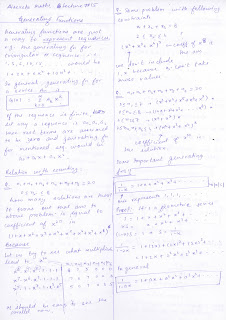## Tuesday, September 29, 2009

### Discrete Maths, Lecture-14 - Discrete Probability

These are my notes from 14th lecture of discrete mathematics course taught at Arsdigita university by Shai Simonson. Other posts relating to this are here.

This lecture introduces basic discrete probability, mainly the two things..

1. P(A) = #of favorable cases where A happen / total # of cases

2. Probability of A, given B has happened

P(A|B) = P(A /\ B) / P(B)

Here are my notes...## Saturday, September 26, 2009

### Discrete Maths, ProblemSet#5

These are my solutions to 5th problem set of discrete mathematics course taught at Arsdigita university by Shai Simonson. Other posts relating to this are here.

Doing this problem set makes me realize that One needs to continually practice solving counting problems to learn permutation-combination properly by heart, it really becomes confusing pretty quickly for not-so-trivial cases.

Anyway, Here are my solutions...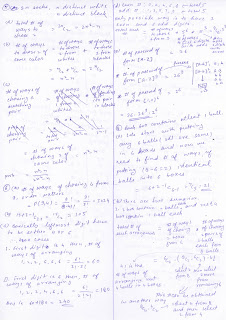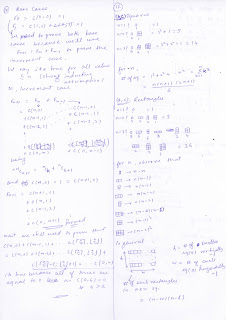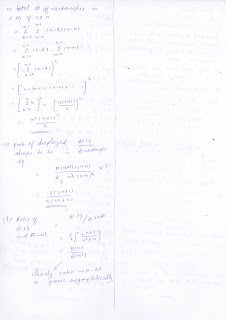### anonymous class in scala

Scala also supports java style syntax of defining anonymous classes. Take a look at this code snippet from Actor companion object definition in Actor.scala from scala actors library.
`  /* Notice the argument of actor method, its a   * no-arg(not empty arg list)   * function that returns Unit */  def actor(body: => Unit): Actor = {    /* Here java style syntax of anonymous classes is being used.     * we're creating an instance not of Actor class but a anonymous     * subclass that is implementing a method act() which is declared     * in trait Reactor(also its evident that its not mandatory to use     * keyword override when implementing something from the trait)     * and overriding scheduler. */    val a = new Actor {      def act() = body      override final val scheduler: IScheduler = parentScheduler    }    /* also see that actor is started right here. */    a.start()    a  }`

### type param in method name in scala

Type parameters can be specified with methods also like in the example below...
`scala> def A[a](b:a){ println(b) }A: [a](a)Unitscala> A[String]("hi")hiscala> A[String](2):6: error: type mismatch; found : Int(2) required: String A[String](2) ^`
They're being used in receive method in Actor class, lets take a look at the signature of receive
`def receive[R](f: PartialFunction[Any, R]):R`
The advantage is that we automatically get the type checking done to be sure that return type of receive is gonna be same as the second type parameter of the PartialFunction.

## Tuesday, September 22, 2009

### Discrete Maths, Lecture-13 : Counting-III

These are my notes from 13th lecture of discrete mathematics course taught at Arsdigita university by Shai Simonson. Other posts relating to this are here.

This lecture covers following topics..
1. Inclusion-Exclusion principle (a generalization of sum rule using same theorem from set theory)
2. Pigeonhole Principle
3. Derrangement Problem

Here are my notes...## Friday, September 18, 2009

### Discrete Maths, Lecture-12 : Counting-II

These are my notes from 12th lecture of discrete mathematics course taught at Arsdigita university by Shai Simonson. Other posts relating to this are here.

This lecture covers generalized permutation and combinations (that basically means repetition is allowed). Though there are "formulae" presented for each case but more emphasis is(and should be) given to how we can think and solve the problems of generalized permutation and combinations using the already covered 5 principles and simple permutation/combination. The intention of this lecture is not to give you the formula but to teach you how you reach to them.

Here are my notes...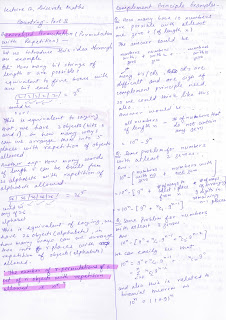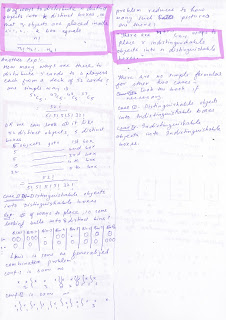## Thursday, September 17, 2009

### combination formula and recursion

Today, while solving some problems in counting I tried to relate the combination formula and recursion.
The idea is, can we derive combination formula using recursion?

How many ways can we make groups of 2 out of n people?

Let T(n) be the answer for n people.
Let us think wishfully and see if we can get to solution for n when solution for n-1 is known.

 [n] [# of pairs] [pairs] 2 - A,B 1 (A,B) 3 - A,B,C 3 (A,B);(B,C);(C,A) 4 - A,B,C,D 6 (A,B);(B,C);(C,D);(D,A);(A,C);(B,D)

From above table, it is easy to observe that
T(n) = T(n-1) + (n-1)
We can solve this recurrence using substitution and see that
T(n) = n(n-1)/2
which is indeed (n combination 2).

How many ways can we make group of k ppl out of n ppl?

Let it be denoted by T(n,k).
Clearly, T(k,k) = 1
We can apply the process as in above case by solving for T(n,3), T(n,4) etc and easily see that
T(n,k) = T(n-1, k) + T(n-1, k-1)

## Wednesday, September 16, 2009

### Discrete Maths, Lecture-11 : Counting-I

These are my notes from 11th lecture of discrete mathematics course taught at Arsdigita university by Shai Simonson. Other posts relating to this are here.

In Summary, this lecture covers following things...
• 5 important principles of counting(2 official and 3 unofficial ones) namely Multiplication principle, Addition principle, Counting the Opposite, Counting double and Just another principle.
• Permutation and Combination(and its relation to Binomial coefficients)
• Interesting observations of Pascal's triangle
• Ways to prove theorems using counting arguments.
A general thing that always helped is that when you're solving some general case n, observe the behaviour for small values of n such as 1,2,3 etc .. this gives good insight/understanding into the problem.

Here are my notes....
Note: Unless specified otherwise, in these notes n objects implicitly mean n distinct objects.## Tuesday, September 15, 2009

### SICP Constraint Network Impl code in Scala

I wrote the code for digital circuit simulator from SICP to contrast my scala coding style with that of Martin Odersky(he wrote same in his book "Programming in Scala"). These are the two(part-I, part-II) posts about that.

To enhance my learnings and to write some more code, Now I implemented the constraint propagation network from SICP section-3.3.5 too.

Here is the code...
`//all the Constraint implementations//extend the Constraint classabstract class Constraint {  def informAboutValue()  def informAboutNoValue()}//Connector implementationclass Connector {  private var myName = "Unknown"  private var myVal:Int = _  private var informant:AnyRef = _  private var constraints:List[Constraint] = List()  def this(newName:String) {    this()    myName = newName  }  def name = myName  def hasValue() = informant != null  def value = {    if(!hasValue())      throw new RuntimeException("""|This connector                                 | does not have a                                 | value.""".stripMargin)    myVal  }  def setValue(newVal:Int, informer:AnyRef) {    Tuple(hasValue(),newVal == myVal) match {      case Tuple2(false, _) => {        myVal = newVal        informant = informer        constraints.foreach(          (c:Constraint) =>             { if(c ne informer) c.informAboutValue()})      }      case Tuple2(true,true) => ; //ignore      case Tuple2(true,false) =>        throw new RuntimeException("Contradiction " +                                   newVal + " " + myVal)    }  }  def forgetValue(retractor:AnyRef) {    if(retractor eq informant) {      informant = null      constraints.foreach(        (c:Constraint) =>           { if(c ne retractor) c.informAboutNoValue() })    }  }  def connect(c:Constraint) {    constraints = c :: constraints  }}//===== Constraints =====case class Adder(a:Connector,b:Connector,                 c:Connector) extends Constraint {  a.connect(this)  b.connect(this)  c.connect(this)  def informAboutValue() {    Tuple(a.hasValue(),b.hasValue(),c.hasValue())     match {      case Tuple3(true,true,false) =>         c.setValue(a.value + b.value, this)      case Tuple3(true,false,true) =>        b.setValue(c.value - a.value, this)      case Tuple3(false,true,true) =>        a.setValue(c.value - b.value, this)      case _ => ; //ignore    }  }  def informAboutNoValue() {    a.forgetValue(this)    b.forgetValue(this)    c.forgetValue(this)    informAboutValue()  }}case class Multiplier(a:Connector,b:Connector,                      c:Connector) extends Constraint {  a.connect(this)  b.connect(this)  c.connect(this)  def informAboutValue() {    Tuple(a.hasValue(),b.hasValue(),          (a.hasValue() && a.value == 0) ||          (b.hasValue() && b.value == 0),          c.hasValue())     match {      case Tuple4(_,_,true,_) =>        c.setValue(0, this)      case Tuple4(true,true,_,false) =>        c.setValue(a.value * b.value, this)      case Tuple4(true,false,_,true) =>        b.setValue(c.value / a.value, this)      case Tuple4(false,true,_,true) =>        a.setValue(c.value / b.value, this)      case _ => ; //ignore    }  }  def informAboutNoValue() {    a.forgetValue(this)    b.forgetValue(this)    c.forgetValue(this)    informAboutValue()  }}case class Constant(value:Int,                    c:Connector) extends Constraint {  c.connect(this)  c.setValue(value, this)  def informAboutValue() {    throw new RuntimeException("""|CONSTANT constraint,                               | request not allowed                               |.""".stripMargin) }  def informAboutNoValue() {    throw new RuntimeException("""|CONSTANT constraint,                               | request not allowed                               |.""".stripMargin) }}case class Probe(c:Connector) extends Constraint {  c.connect(this)  def informAboutValue() {    printProbe(c.value.toString()) }  def informAboutNoValue() {    printProbe("?") }  private def printProbe(value:String) {    println("Probe: " + c.name + " = " + value)  }}//====== simulation =====def celsiusFahrenheitConverter(c:Connector,f:Connector) {  val u = new Connector()  val v = new Connector()  val w = new Connector()  val x = new Connector()  val y = new Connector()  Multiplier(c, w, u)  Multiplier(v, x, u)  Adder(v, y, f)  Constant(9, w)  Constant(5, x)  Constant(32, y)}val C = new Connector("Celsius Temp")Probe(C)val F = new Connector("Fahrenheit Temp")Probe(F)celsiusFahrenheitConverter(C,F)C.setValue(25, 'user)//Probe: Fahrenheit Temp = 77//Probe: Celsius Temp = 25F.setValue(212, 'user)//java.lang.RuntimeException: Contradiction 212 77C.forgetValue('user)//Probe: Fahrenheit Temp = ?//Probe: Celsius Temp = ?F.setValue(212, 'user)//Probe: Celsius Temp = 100//Probe: Fahrenheit Temp = 212`

## Monday, September 14, 2009

### SICP digital circuit simulator in scala Part II

Here I am comparing the differences between my version of the simulator and Martin Odersky's version presented in his book "Programming in Scala" in chapter-18(Stateful Objects).

Overall, It is same as my version(we both are porting the same thing from same source :)). But, yet there are some differences worth noticing..

1. Inside the Simulation class(in my code its the Agenda class), he defines a type
type Action = ()=>Unit
What this does is that it creates an alias Action for the type procedure that takes no arguments and returns Unit. Now he can use Action instead of ()=>Unit everywhere and this enhances readability of the code. I did not know of this concept because its covered in a later chapter(20) in the book and I'm at chapter 18 :).

2. Another trivial difference, He made afterDelay procedure a part of Simulation class itself... I could have done same and its better.

3. He used currying in afterDelay so as to make the calls look as if its a built-in syntax. I don't agree with it... As a code reader I would not want to be confused into understanding a method call as language syntax. But, may be, As I get more experienced with this concept... I'll probably change my stand.

4. Trivial, but he exposes the usage of @unchecked in the match expression when getting next item on the agenda to suppress the warning that case set is not exhaustive as we no for sure that the missing case in question is never gonna happen.

5. Whenever I wrote scala classes and there was a collection(let say List) to be stored, I had two choices...
val l:mutableList
var l:immutableList
Now here is the conflict.. functional style says
- Prefer val over var
- Prefer immutable object over mutable object
As you can see, We can't have both here.
In the Wire class, author choses the second approach of using immutable object with var reference for storing actions. Incidentally I did the same in my code, I was not sure about my decision at the time of doing it but seeing him making the same choice tells me which rule to give preference to in case of conflict :).
However, The reason I chose immutable object was that I felt if its a mutable object and my reference(actions) is exposed publicly, anyone can get hold of it and modify the object(actions List) without Wire knowing about it.

6. The most visible(and important) difference is in the way how he managed agenda items. I implemented it exactly same as in SICP by making Segment(a pair of time, Queue) stored in a priority queue. But, he simplified it. He stored items simply in a single list making sure a new item with smaller(not matching any other item time on the list) time is added before the next later time item in the list. And, a new item is added in the end of other items added for the same time so that they are accessed in FIFO order. Now, accessing the items is so simplified.

7. For constants like delays, he uses the naming convention(described in the book also) of keeping first letter capital as in ConstantVariable.

The biggest learning for me(from point 6), I guess, is that when I port... I more or less map the code from one language to another without changing any implementation detail conceptually. I should actually see if the some implementation detail can be simplified due to new target language features or simply because it can be simplified.

## Sunday, September 13, 2009

### SICP digital circuit simulator in scala Part I

This is a scala port of digital circuit simulator of section 3.3.4 in SICP. Martin Odersky has done same in Chapter-18(Stateful Objects) in his book Programming in Scala. Before reading his code, I wanted to write it on my own so that I can understand where I don't think *in scala* when writing code in Scala.
Next, I'll read the same port from mentioned scala book and see what I can learn from the differences.

Here is my code(I tested it on Scala version 2.7.5.final interpreter)...
`//Segment and Agendaimport scala.collection.mutable.Queuecase class Segment(val time:Int,     val queue:Queue[()=>Unit]) extends Ordered[Segment] {  require(time >= 0)    override def compare(that:Segment) = {    if(that.time == time) 0    else if(that.time < time) -1    else 1  }}import scala.collection.mutable.PriorityQueueclass Agenda {  private var currentTime = 0  private val timeSegments = new PriorityQueue[Segment]()  def getCurrentTime = currentTime  def addItem(time:Int, item:()=>Unit) {    getTimeSegment(time) match {      case None =>          val queue = new Queue[()=>Unit]()        queue += item        timeSegments += Segment(time, queue)      case Some(segment) => segment.queue += item    }  }  def propagate():Unit = {    if(!timeSegments.isEmpty) {      firstItem()      propagate()    }     else println("Agenda Propagation Done.")  }  //gets first agenda item and removes it  //from the agenda  private def firstItem = {    val segment = timeSegments.max    currentTime = segment.time        val item = segment.queue.dequeue    if(segment.queue.isEmpty)      timeSegments.dequeue        item  }  //get the Segment matching time from timeSegments  private def getTimeSegment(time:Int) = {    val tmp = timeSegments.filter((s:Segment)=>s.time == time)    if(tmp.isEmpty) None else Some(tmp.first)  }}//Wireclass Wire() {  var signal = false  private var actions:List[()=>Unit] = List()  def setSignal(b:Boolean) {    if(b != signal) {      signal = b      //execute all actions      actions.foreach((proc:()=>Unit)  => proc())    }  }  def addAction(proc:()=>Unit) {     actions = proc :: actions     proc()  }}//Probedef probe(name:String, wire:Wire, agenda:Agenda) {  wire.addAction(    () =>      println(name + " " + agenda.getCurrentTime               + " ,New Value = " + wire.signal))}//after-delaydef afterDelay(delay:Int, proc:() => Unit, agenda:Agenda) {  require(delay >= 0)  agenda.addItem(agenda.getCurrentTime + delay, proc)}//====================== SIMULATION ========================val theAgenda = new Agenda()val inverterDelay = 2val andGateDelay = 3val orGateDelay = 5//inverterdef inverter(in:Wire, out:Wire) = {  in.addAction(    () => {       val newVal = !in.signal      afterDelay(inverterDelay, () =>         (out.setSignal(newVal)),theAgenda)    })  "Ok"}//and gatedef andGate(in1:Wire, in2:Wire, out:Wire) = {  val action = () => {    val newVal = in1.signal && in2.signal    afterDelay(andGateDelay,               () => (out.setSignal(newVal)),               theAgenda) }  in1.addAction(action)  in2.addAction(action)  "Ok"}//or gatedef orGate(in1:Wire, in2:Wire, out:Wire) = {  val action = () => {    val newVal = in1.signal || in2.signal    afterDelay(orGateDelay,               () => (out.setSignal(newVal)),               theAgenda) }  in1.addAction(action)  in2.addAction(action)  "Ok"}//half-adderdef halfAdder(in1:Wire, in2:Wire, sum:Wire, carry:Wire) = {  val t1 = new Wire()  val t2 = new Wire()  orGate(in1, in2, t1)  andGate(in1, in2, carry)  inverter(carry, t2)  andGate(t1, t2, sum)  "Ok"}// == Running the simulation ==val in1 = new Wire()val in2 = new Wire()val sum = new Wire()val carry = new Wire()probe("sum",sum,theAgenda)//sum 0 ,New Value = falseprobe("carry",carry,theAgenda)//carry 0 ,New Value = falsehalfAdder(in1,in2,sum,carry)//Okin1.setSignal(true)theAgenda.propagate()//sum 8 ,New Value = true//Agenda Propagation Done.in2.setSignal(true)theAgenda.propagate()//carry 11 ,New Value = true//sum 16 ,New Value = false//Agenda Propagation Done.`

## Thursday, September 10, 2009

### Introduction to the term "ORM"

In enterprise applications we have many objects that we want to be persistent, i.e. we want their existence beyond system restarts. For it to happen one should be able to store the object state to some persistent storage and reconstruct the object from the state whenever needed. One object is a simple case, Its very usual that we need to store a whole object graph that we want to recover later.

Relational databases have been used to store data, and the technology is mature, hence its the natural choice for storing object state in relational databases.

Relational databases expose SQL based API for interaction. In java, one can use JDBC to create SQL queries, bind arguments to parameters, initiate execution of the query, scroll through the query results, retrieve values from the result-set and so on. These are very low level data access tasks; and as application developer one is more interested in solving the business problem rather than data access. So came the concept of persistence layer which should abstract all the details of data access and provide an API to business layer to deal just with the objects and not with low level stuff such as result-sets.

Let us look at some of the problems that the persistence layer faces due to paradigm mismatch between the object view and relational view of the world.

The Problem of Granularity:
If one object contains another object of different type(one and only one instance, noone else contains objects of this type). Should we create two different tables for both types or just one table having columns that take care of columns from both.

The Problem of subtypes:
How do we model inheritance in relational database.

The problem of associations:
How do we model one-to-many, many-to-many relationships.

The problem of object graph navigation:
Abstracting out the details of data access so that biz layer can navigate objects graphs in natural object oriented way like aUser.getBillingDetails().getAcoountNumber() rather than using SQL join queries.

If the application is simple enough then we can model our system relationally or may handcode the persistence layer with SQL/JDBC. Other solutions are serialization, EJB entity beans or using object-oriented database systems rather than relational databases.

ORM(Object-Relational mapper) is an attempt to create a generic piece of software that can take care of all the persistence related issues mentioned above.
It is the automated(and trasparent) persistence of objects in an application to the tables in a relational database, using metadata that describes the mapping between the objects and the database tables. ORM is supposed to provide atleast following things..
• An API for performing basic CRUD operations on objects of persistent classes.
• A query language based on the persistence classes and their properties.
• Facilities for specifying mapping metadata.
• Various optimization facilities like lazy association fetching, caching, automatic syncing of object state with same in database.
For an application that has to scale, even above is not sufficient. One also needs to think about multi-tenancy and partitioning.

(Potential)Advantages of Using ORM: Productivity, Maintainability, Performance and Vendor Independence

Reference: Chapter 1, Hibernate in Action